Question

# An organ pipe is observed to produce 3 consecutive harmonics with frequencies of 189, 243 and...

An organ pipe is observed to produce 3 consecutive harmonics with frequencies of 189, 243 and 297 Hz. By how much does the tube need to be shortened so that the fundamental frequency is 60 Hz? The speed of sound is 343 m/s.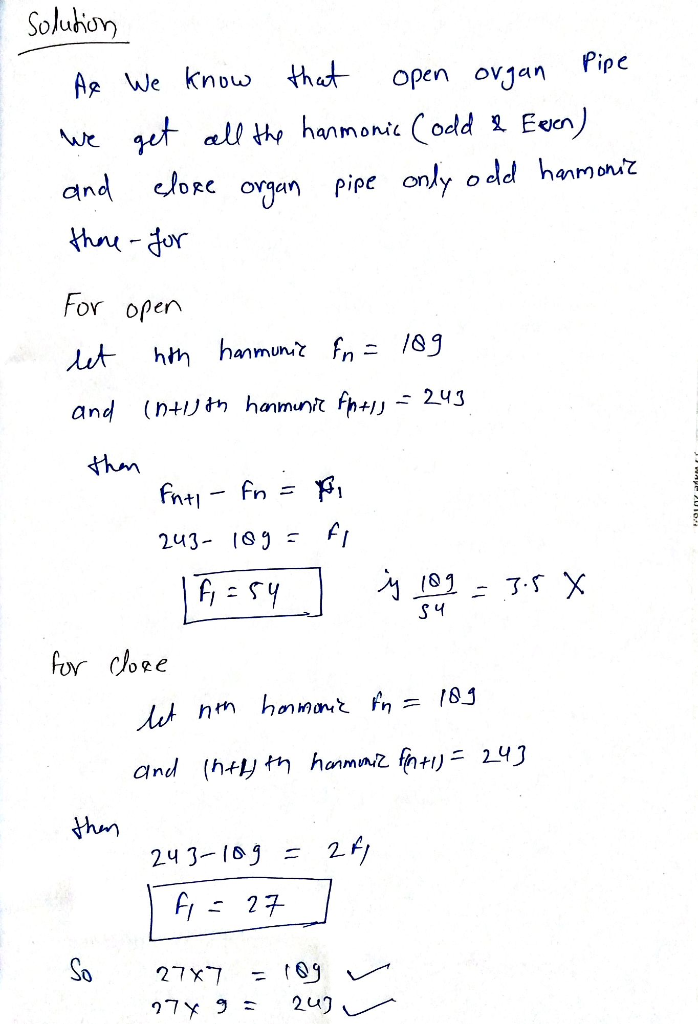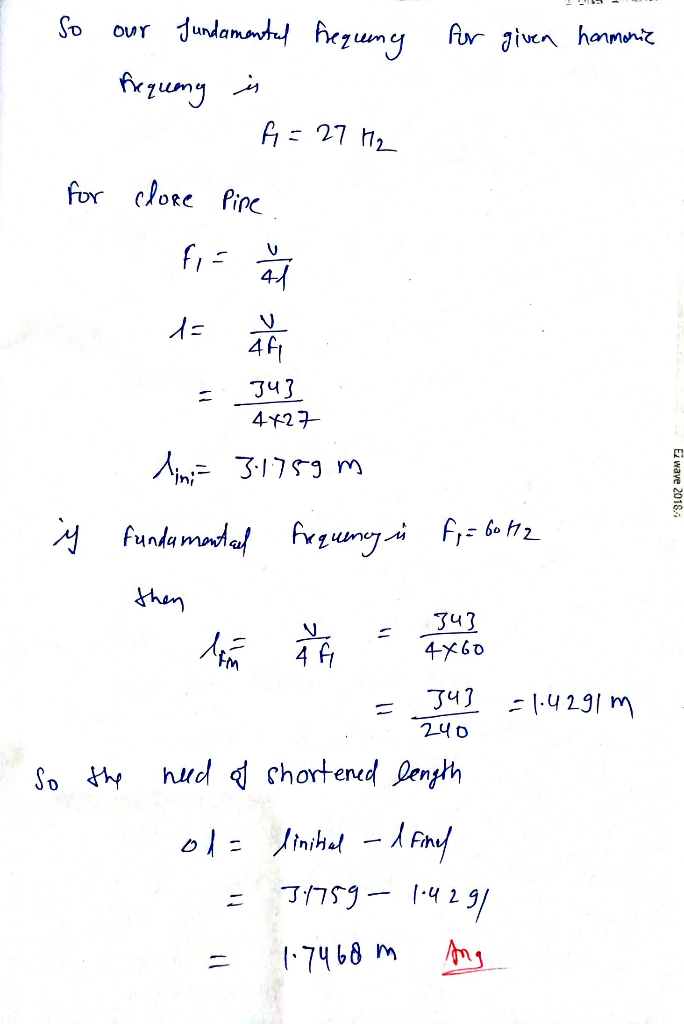#### Earn Coins

Coins can be redeemed for fabulous gifts.

Similar Homework Help Questions
• ### frequencies of an organ pipe are determined to be 702 Hz and 810 HE. (Assume the...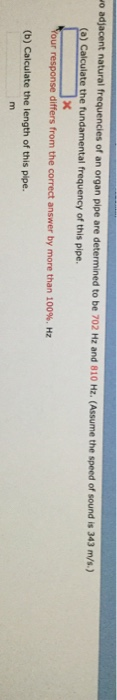frequencies of an organ pipe are determined to be 702 Hz and 810 HE. (Assume the speed of sound is 343 m/s.) a) Calculate the fundamental frequency of this pipe xt our response differs from the correct answer by more than 100%. Hz (b) Calculate the length of this pipe.

• ### 6)Two adjacent natural frequencies of an organ pipe are determined to be 912 Hz and 1008...

6)Two adjacent natural frequencies of an organ pipe are determined to be 912 Hz and 1008 Hz. (Assume the speed of sound is 343 m/s.) (a) Calculate the fundamental frequency of this pipe. Answer must be in Hz (b) Calculate the length of this pipe. Answer must be in m 7)A train sounds its horn as it approaches an intersection. The horn can just be heard at a level of 60 dB by an observer 10 km away. (a) What...

• ### SOLUTION (A) Find the frequencies if the pipe is open at both ends. _V 343 m/s...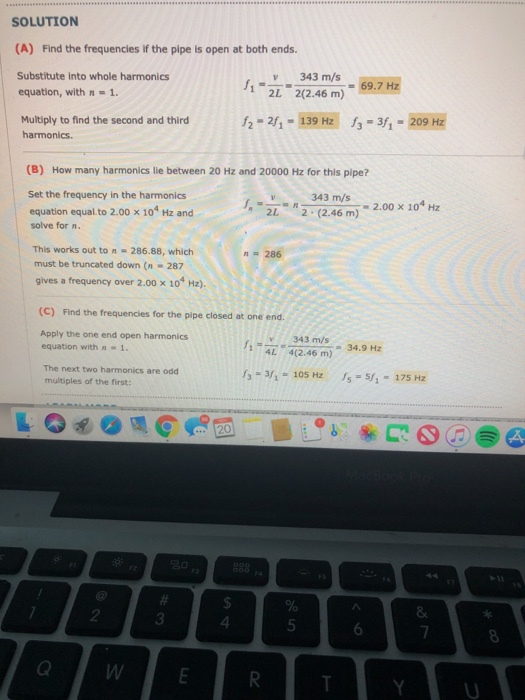SOLUTION (A) Find the frequencies if the pipe is open at both ends. _V 343 m/s Substitute into whole harmonics equation, with n = 1. 11-222(2.46 m) = 69.7 Hz Multiply to find the second and third harmonics. 12 - 27 - 139 Hz 13 = 3f7 - 209 Hz (B) How many harmonics lle between 20 Hz and 20000 Hz for this pipe? 343 m/s Set the frequency in the harmonics equation equal to 2.00 x 104 Hz and...

• ### Question 1 Atube with one end open and one end closed creates two consecutive harmonic frequencies...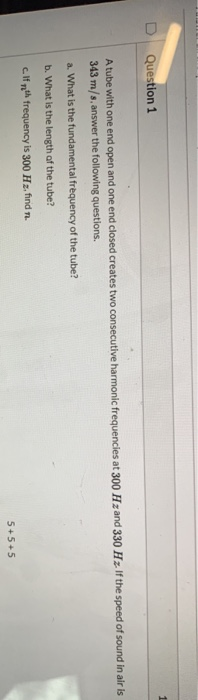Question 1 Atube with one end open and one end closed creates two consecutive harmonic frequencies at 300 Hz and 330 Hz. If the speed of sound in air is 343 m/s, answer the following questions. a. What is the fundamental frequency of the tube? b. What is the length of the tube? c. If mith frequency is 300 Hz, find 91. 5+5+5

• ### Organ pipe A with both ends open has a fundamental frequency of 320.0 Hz. The third...

Organ pipe A with both ends open has a fundamental frequency of 320.0 Hz. The third harmonic of organ pipe B with one end open has the same frequency as the second harmonic of pipe A. Assume a speed of sound of 343 m/s. What is the length of Pipe A? What is the length of Pipe B?

• ### A particular tube for a pipe organ is 4m long and open at both ends. The speed of sound is about 340m/s. Draw the fi...

A particular tube for a pipe organ is 4m long and open at both ends. The speed of sound is about 340m/s. Draw the first three harmonics and find the frequencies for the pressure wave view of sound. For each frequency, find another tube length that could also have this frequency as a harmonic. Now pretend the tube is closed at one end. Draw the first two harmonics and find the frequencies.

• ### A 146-cm-long pipe is stopped at one end. Near the open end, there is a loudspeaker...

A 146-cm-long pipe is stopped at one end. Near the open end, there is a loudspeaker that is driven by an audio oscillator whose frequency can be varied from 10.0 to 4700 Hz. (Take the speed of sound to be 343 m/s.) (a) What is the lowest frequency of the oscillator that will produce resonance within the tube?   Hz (b) What is the highest frequency that will produce resonance?   Hz (c) How many different frequencies of the oscillator will produce...

• ### A tube, open at the left end and closed at the right, has standing-wave patterns at...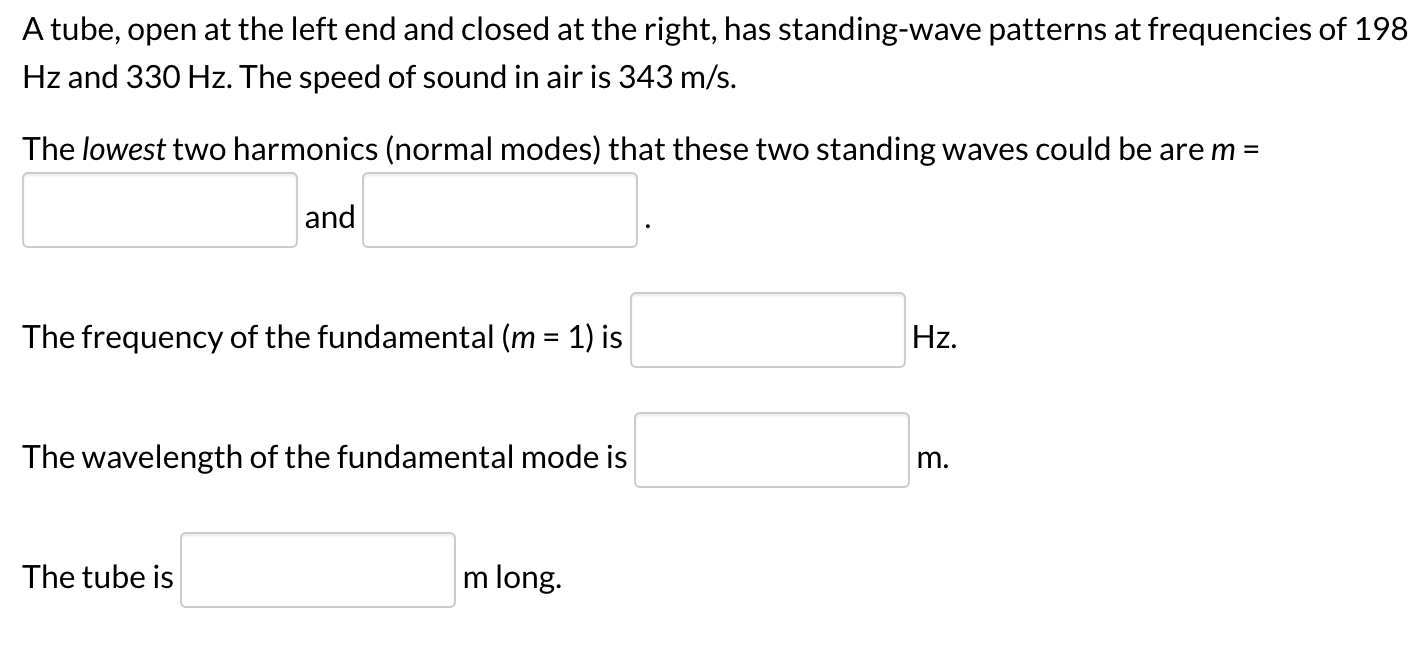A tube, open at the left end and closed at the right, has standing-wave patterns at frequencies of 198 Hz and 330 Hz. The speed of sound in air is 343 m/s. The lowest two harmonics (normal modes) that these two standing waves could be are m = and The frequency of the fundamental (m = 1) is Hz. The wavelength of the fundamental mode is m. The tube is m long

• ### Calculate the length of a pipe that has a fundamental frequency of 316 Hz. (Take the...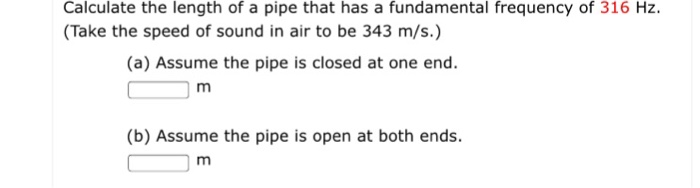Calculate the length of a pipe that has a fundamental frequency of 316 Hz. (Take the speed of sound in air to be 343 m/s.) Calculate the length of a pipe that has a fundamental frequency of 316 Hz. (Take the speed of sound in air to be 343 m/s.) (a) Assume the pipe is closed at one end (b) Assume the pipe is open at both ends

• ### A tuba may be treated like a tube closed at one end. If a tuba has...

A tuba may be treated like a tube closed at one end. If a tuba has a fundamental frequency of 90.9 Hz, determine the first three overtones. Use 343 m/s as the speed of sound in air. first overtone        How is the length of a tube closed at one end related to the resonant wavelengths that can be established in the tube? How are the frequency, wavelength, and speed of sound related? How are the harmonics related to the...